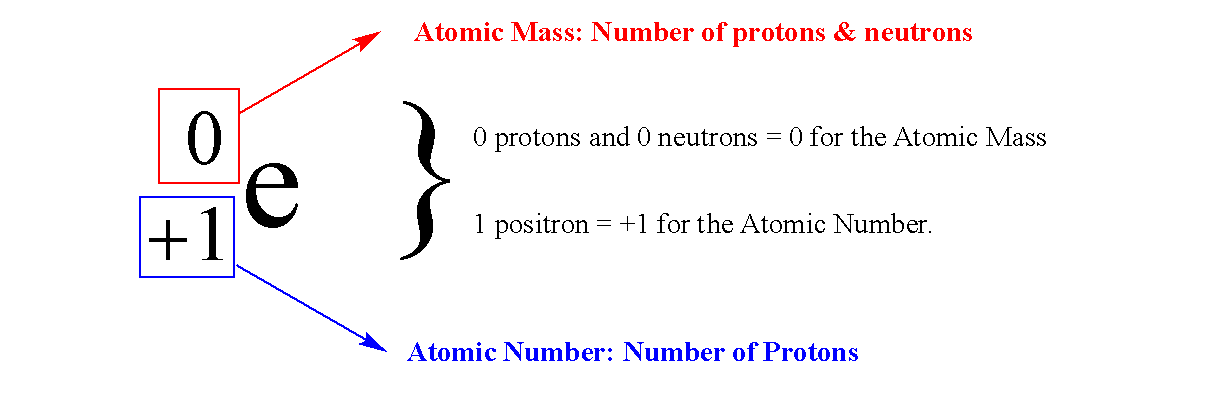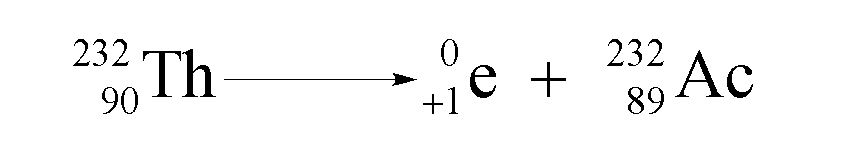Ch.11 Nuclear ChemistryWorksheetSee all chapters

# Positron Emission

See all sections
Sections
Alpha Decay
Beta Decay
Gamma Emission
Electron Capture
Positron Emission
Decay Series
Stability of Isotopes
Nuclear Transmutation
Mass Defect

A positron emission or positron decay occurs when an unstable nucleus ejects a positron particle to create a new element.

###### Understanding Positron Emission

A positron particle is referred to as the anti-electron particle because it looks like a positively charged electron.Concept #1: Understanding Positron Emission

###### Expert Q&A

Ask unlimited questions and get expert help right away.

Transcript

Hey guys! In this new video, we’re going to take a look at positron emission. Here, we're going to say positron emission occurs when an unstable nucleus emits a positron.
What's a positron? A positron is an antiparticle of the electron. Remember, the electron is represented by this. A positron is the opposite of that. It looks like an electron but instead of it having a negative sign, it'll have the opposite sign. It will be a positive electron. A positron is considered just a positive electron. I know this is weird but again, remember we're dealing with nuclear reactions. A lot of unaccustomed things that we are not used to seeing do occur. One of them is this positron. We’re going to say here, here’s our positron. Because we're talking about the word emission again, emission would mean decay which means that this positron would be a product.
Let’s think of an example. Here, Einstein has his own element named after him, Einsteinium. We’ll deal with isotope 253 of Einsteinium. Einsteinium is Es on our periodic table. It has an atomic number of 99. We're going to emit a positron and because we're emitting a positron, let’s see. Because the atomic mass is 0, the new element is still going to be 253. But because the bottom is one, what number plus 1 gives me 99? It’d have to be a 98. Here, that would just be Cf. This would be an example of a positron decay or positron emission.Example #1: Write balanced nuclear equations for each of the following positron emissions.

a) Uranium (U) – 235

###### Expert Q&A

Ask unlimited questions and get expert help right away.

Transcript

Based on that, let's answer these two questions. It says: Write balanced nuclear equations for each of the following positron emissions. Again, your positron will be a product. Here we're dealing with uranium 235. Uranium is U. On your periodic table, it's going to have an atomic number of 92. We emit a positron so this is going to be 235 and 91 plus 1, it gives me 92. 91 would be in Pa.
Next one is radon which is Rn. Radon has an atomic number of 86. We emit a positron so now the element is still going to be 222 and 85 plus 1 gives me 86. That's actinium. These would be two examples of our positron emission.

Example #2: A nuclide of Th-225 undergoes 3 alpha decays, 4 beta decays, and a gamma emission. What is the product?